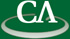## Algebro-Geometric Aspects of Analytic Combinatorics in Several Variables.

### Thursday May 25rd at 11:30am in K9509.

```Abstract

In the study of enumeration, a standard method of deriving asymptotic
information about a sequence of interest is to study analytic properties of
its generating function.  In many interesting applications, however, one
does not have access to the univariate generating function directly but
knows it as a sub-series of the multivariate power series expansion of a
rational function in several variables.  Trying to determine asymptotic
information from this less direct representation is the purpose of the new
field of Analytic Combinatorics in Several Variables (ACSV), which raises
many interesting questions across several domains of mathematics.

This talk will discuss the algebro-geometric problems which arise, and
detail the first rigorous algorithms implementing the methods of ACSV under
assumptions which are often satisfied in practice. This work combines
effective versions of the arithmetic Nullstellensatz with tools from
polynomial system solving dating back to Kronecker to design rigorous
symbolic-numeric algorithms.  Genericity of the required assumptions is
proven through the use of the multivariate resultant.
```